# Uniquely colorable perfect graphs

Uniquely colorable perfect graphs (in which there is a unique partition into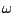stable-sets) are closely related to minimal imperfect graphs: according to a result of Padberg (Perfect zero-one matrices, Math Programming, 6, (1974)) if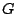is minimal imperfect then for all of its vertices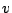, the graph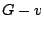is uniquely colorable.

There is also a combinatorial good characterization theorem for unique colorability of perfect graphs, and a polynomial algorithm for testing the property using the ellipsoid method (IPCO 1, Kannan, Pulleyblank eds, Waterloo Univ. Press, 1990). In other words UNIQUE COLORABILITY is a tractable property for perfect graphs, closely related to minimal imperfect graphs.

Yet, some simple conjectures related to the SPGT, resist through the years. The following one arises both by specializing more general conjectures occurring in various papers, and does not seem to trivially follow from the SPGT:

If G is perfect and uniquely colorable, does there exist two-cliques which meet in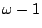points ?

Let us call the two vertices in the symmetric difference of two such cliques forced.

Is it true that every known uniquely colorable perfect graph collapses to an-clique by successive identification of forced vertices ?

It can be simply proved that a minimal imperfect graph with three forced vertices in particular positions is an odd hole or an odd antihole. A simpler proof of the following statement would shortcut the proof of the SPGT:

If G is minimal imperfect, there is a vertexso that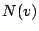is uniquely colorable.

Contributed by Jean Fonlupt and András Sebo

Back to the main index for Perfect Graphs.